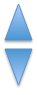# Common Core: 1st Grade Math : Compose Two-Dimensional or Three-Dimensional Shapes to Create a Composite Shape: CCSS.MATH.CONTENT.1.G.A.2

## Example Questions

← Previous 1

### Example Question #1 : Compose Two Dimensional Or Three Dimensional Shapes To Create A Composite Shape: Ccss.Math.Content.1.G.A.2

What two shapes can you find in this shape?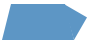A rectangle and a triangle

A trapezoid and a square

A trapezoid and a triangle

A square and a triangle

A trapezoid and a triangle

Explanation: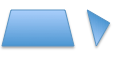### Example Question #1 : Compose Two Dimensional Or Three Dimensional Shapes To Create A Composite Shape: Ccss.Math.Content.1.G.A.2

What two shapes can you find in this shape?A square and a triangle

A square and a trapezoid

A square and a square

A triangle and a trapezoid

A square and a square

Explanation: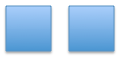### Example Question #2 : Compose Two Dimensional Or Three Dimensional Shapes To Create A Composite Shape: Ccss.Math.Content.1.G.A.2

What two shapes can you find in this shape?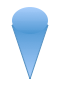A triangle and a circle

A rectangle and a circle

A square and a circle

A heptagon and a circle

A triangle and a circle

Explanation: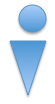### Example Question #3 : Compose Two Dimensional Or Three Dimensional Shapes To Create A Composite Shape: Ccss.Math.Content.1.G.A.2

What two shapes can you find in this shape?A square and a rectangle

A square and a triangle

A square and a trapezoid

A square and a circle

A square and a trapezoid

Explanation: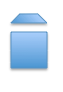### Example Question #93 : Quadrilaterals

What two shapes can you find in this shape?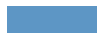A rectangle and a trapezoid

A rectangle and a square

A square and a triangle

A rectangle and a triangle

A rectangle and a square

Explanation: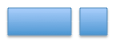### Example Question #4 : Compose Two Dimensional Or Three Dimensional Shapes To Create A Composite Shape: Ccss.Math.Content.1.G.A.2

What two shapes can you find in this shape?A cube and a trapezoid

A cube and a triangle

A cube and a square

A cube and a circle

A cube and a square

Explanation: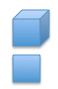### Example Question #31 : Reason With Shapes And Their Attributes

What two shapes can you find in this shape?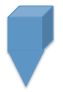A cube and a rectangle

A cube and a triangle

A cube and a square

A cube and a trapezoid

A cube and a triangle

Explanation: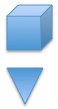### Example Question #1 : Compose Two Dimensional Or Three Dimensional Shapes To Create A Composite Shape: Ccss.Math.Content.1.G.A.2

What two shapes can you find in this shape?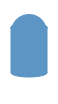A cylinder and a triangle

A rectangle and a triangle

A rectangle and circle

A cylinder and a circle

A cylinder and a circle

Explanation: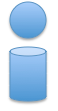### Example Question #3 : Compose Two Dimensional Or Three Dimensional Shapes To Create A Composite Shape: Ccss.Math.Content.1.G.A.2

What two shapes do you see in this shape?A triangle and a hexagon

A pentagon and a rectangle

A triangle and a pentagon

A pentagon and a square

A triangle and a pentagon

Explanation:### Example Question #1 : Compose Two Dimensional Or Three Dimensional Shapes To Create A Composite Shape: Ccss.Math.Content.1.G.A.2

What two shapes can you find in this shape?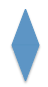A rectangle and a triangle

A triangle and a square

A square and a square

A triangle and a triangle